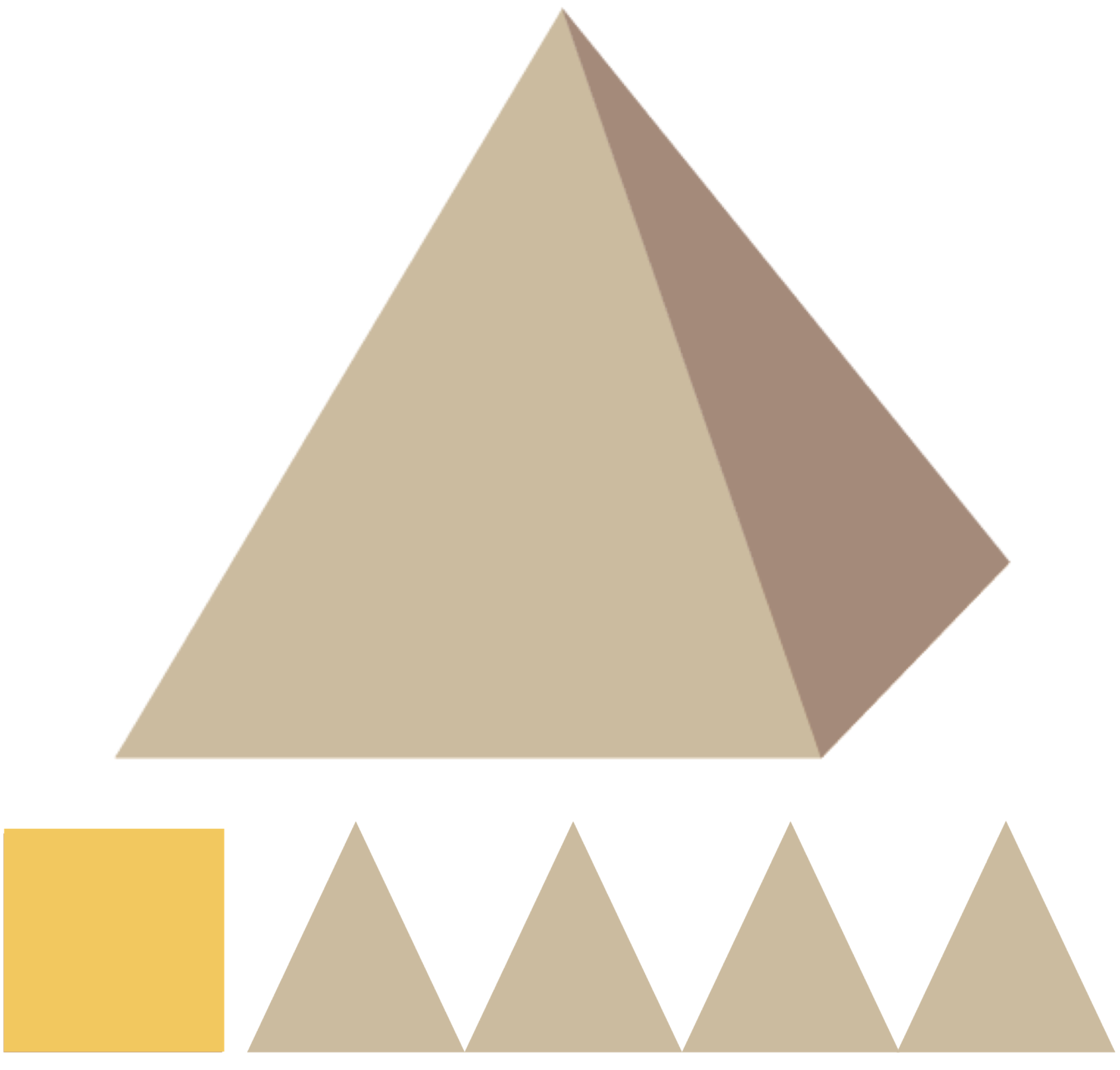# How to Find Volume and Surface Area of a Pyramid

Here you will learn to find the surface area and volume of a pyramid. A pyramid is a geometric figure that has a polygon as its base and as many side faces as the base has sides. The side faces are all triangles whose tops meet in a point.## Surface Area

Rule

### SurfaceAreaofaPyramid

Imagine that the pyramid is unfolded. The surface area is found by adding the area of the base and the areas of all the side faces.

Example 1

Find the surface area of a pyramid where the base is a square with sides of $\text{}5\text{}\phantom{\rule{0.17em}{0ex}}\text{m}$. The height of the side faces of the pyramid is $\text{}6\text{}\phantom{\rule{0.17em}{0ex}}\text{m}$.

First you need to find the area of the base surface:

 ${A}_{b}=5\phantom{\rule{0.17em}{0ex}}\text{m}\cdot 5\phantom{\rule{0.17em}{0ex}}\text{m}=25\phantom{\rule{0.17em}{0ex}}{\text{m}}^{2}$

Then you can find the area of the sides. Each side surface is a triangle with a base equal to $5$ m and a height of $6$ m:

 ${A}_{s}=\frac{5\cdot 6}{2}=15\phantom{\rule{0.17em}{0ex}}{\text{m}}^{2}$

Since the base is a square, that is, a quadrilateral, you have four such triangles. The total surface area then becomes

 $A={A}_{b}+4\cdot {A}_{s}=25\phantom{\rule{0.17em}{0ex}}{\text{m}}^{2}+4\cdot 15\phantom{\rule{0.17em}{0ex}}{\text{m}}^{2}=85\phantom{\rule{0.17em}{0ex}}{\text{m}}^{2}.$

## Volume

Formula

### VolumeofaPyramid

 $V=\frac{B\cdot h}{3},$

where $B$ is the area of the base, and $h$ is the height of the pyramid.

Example 2

What is the volume of a pyramid that has a triangular base, where the triangle has a base of $\text{}4\text{}\phantom{\rule{0.17em}{0ex}}\text{m}$ and a height of $\text{}3\text{}\phantom{\rule{0.17em}{0ex}}\text{m}$? The height of the pyramid is $\text{}5\text{}\phantom{\rule{0.17em}{0ex}}\text{m}$.

First you need to calculate the area of the base: $\begin{array}{llll}\hfill B& =\frac{4\cdot 3}{2}=6\phantom{\rule{0.17em}{0ex}}{\text{m}}^{2}\phantom{\rule{2em}{0ex}}& \hfill & \phantom{\rule{2em}{0ex}}\\ \hfill V& =\frac{6\cdot 5}{3}=10\phantom{\rule{0.17em}{0ex}}{\text{m}}^{3}\phantom{\rule{2em}{0ex}}& \hfill & \phantom{\rule{2em}{0ex}}\end{array}$